﻿ Python: Append the same value /a list multiple times to a list/list-of-lists - w3resource# Python: Append the same value /a list multiple times to a list/list-of-lists

## Python List: Exercise - 159 with Solution

Write a Python program to append the same value /a list multiple times to a list/list-of-lists.

Sample Solution:

Python Code:

``````print("Add a value(7), 5 times, to a list:")
nums = []
nums += 5 * ['7']
print(nums)
nums1 = [1,2,3,4]
print("\nAdd 5, 6 times, to a list:")
nums1 += 6 * 
print(nums1)
print("\nAdd a list, 4 times, to a list of lists:")
nums1 = []
nums1 += 4 * [[1,2,5]]
print(nums1)
print("\nAdd a list, 4 times, to a list of lists:")
nums1 = [[5,6,7]]
nums1 += 4 * [[1,2,5]]
print(nums1)
```
```

Sample Output:

```Add a value(7), 5 times, to a list:
['7', '7', '7', '7', '7']

Add 5, 6 times, to a list:
[1, 2, 3, 4, 5, 5, 5, 5, 5, 5]

Add a list, 4 times, to a list of lists:
[[1, 2, 5], [1, 2, 5], [1, 2, 5], [1, 2, 5]]

Add a list, 4 times, to a list of lists:
[[5, 6, 7], [1, 2, 5], [1, 2, 5], [1, 2, 5], [1, 2, 5]]
```

Pictorial Presentation: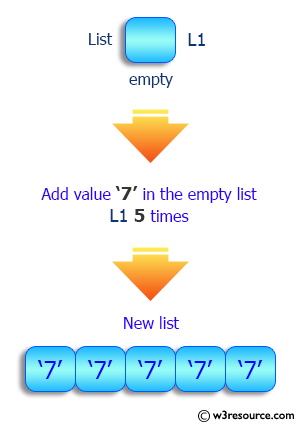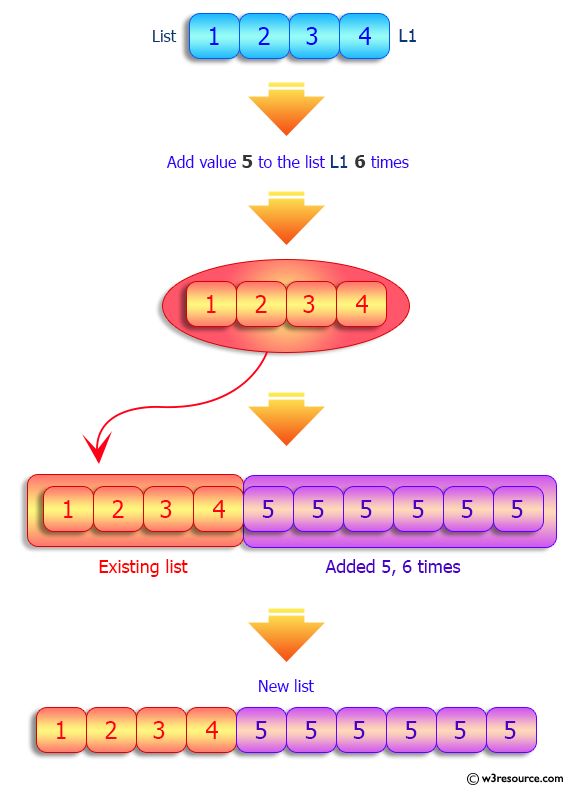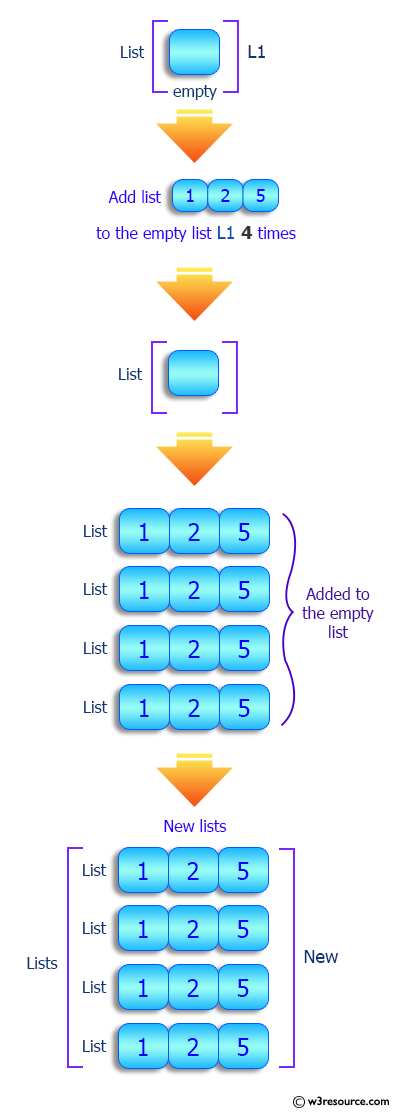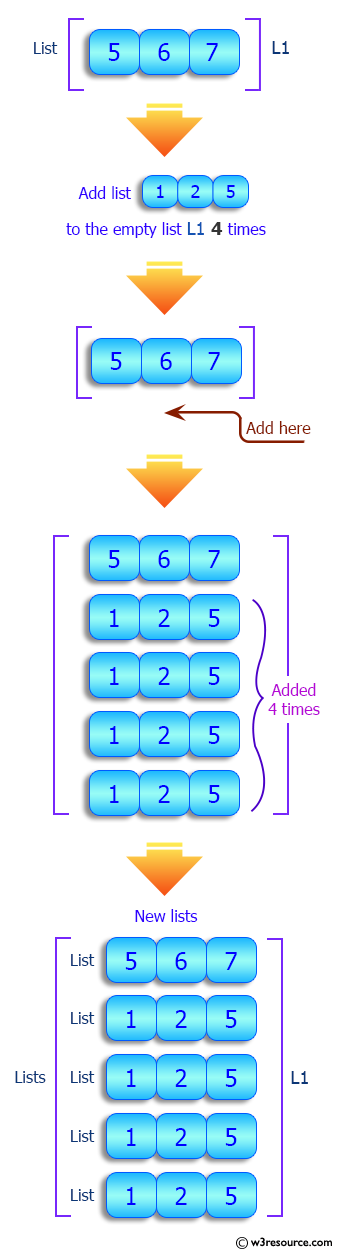Flowchart: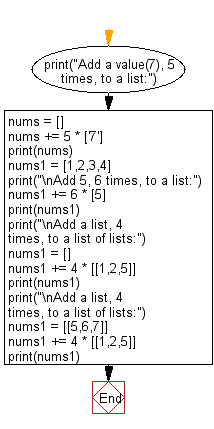## Visualize Python code execution:

The following tool visualize what the computer is doing step-by-step as it executes the said program:

Python Code Editor:

Have another way to solve this solution? Contribute your code (and comments) through Disqus.

What is the difficulty level of this exercise?

Test your Python skills with w3resource's quiz

﻿

## Python: Tips of the Day

Floor Division:

When we speak of division we normally mean (/) float division operator, this will give a precise result in float format with decimals.

For a rounded integer result there is (//) floor division operator in Python. Floor division will only give integer results that are round numbers.

```print(1000 // 300)
print(1000 / 300)```

Output:

```3
3.3333333333333335```